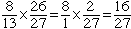Name: Arias Who is asking: Student Level: Middle Question: 8/13*26/27= Hi Arias, The convention for the order of operations when evaluating arithmetic expressions is Operations that are inside Parentheses Powers (Exponents) Division Multiplication Addition Subtraction Some people remember this as PEDMAS. Hence in your expression perform the divisions first then the multiplication, that is find  8/13 and  26/27 and then multiply them together. Hence, since 26 = 2 x 13, you getA mathematician would never write 8/13*26/27. I would write (8/13)*(26/27) so that it is clear which operation to perform first. Penny Go to Math Central